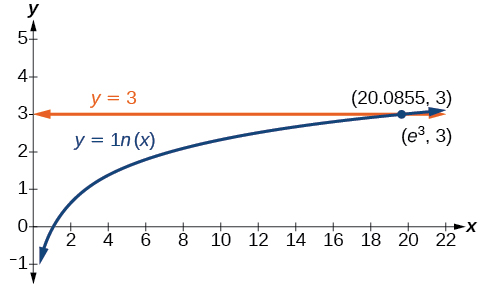# 4.6 Exponential and logarithmic equations  (Page 4/8)

 Page 4 / 8

## Using the definition of a logarithm to solve logarithmic equations

For any algebraic expression $\text{\hspace{0.17em}}S\text{\hspace{0.17em}}$ and real numbers $\text{\hspace{0.17em}}b\text{\hspace{0.17em}}$ and $\text{\hspace{0.17em}}c,$ where

## Using algebra to solve a logarithmic equation

Solve $\text{\hspace{0.17em}}2\mathrm{ln}x+3=7.$

Solve $\text{\hspace{0.17em}}6+\mathrm{ln}x=10.$

$x={e}^{4}$

## Using algebra before and after using the definition of the natural logarithm

Solve $\text{\hspace{0.17em}}2\mathrm{ln}\left(6x\right)=7.$

Solve $\text{\hspace{0.17em}}2\mathrm{ln}\left(x+1\right)=10.$

$x={e}^{5}-1$

## Using a graph to understand the solution to a logarithmic equation

Solve $\text{\hspace{0.17em}}\mathrm{ln}x=3.$

[link] represents the graph of the equation. On the graph, the x -coordinate of the point at which the two graphs intersect is close to 20. In other words $\text{\hspace{0.17em}}{e}^{3}\approx 20.\text{\hspace{0.17em}}$ A calculator gives a better approximation: $\text{\hspace{0.17em}}{e}^{3}\approx 20.0855.$The graphs of   y = ln x   and   y = 3   cross at the point   (e 3 , 3 ) , which is approximately (20.0855, 3).

Use a graphing calculator to estimate the approximate solution to the logarithmic equation $\text{\hspace{0.17em}}{2}^{x}=1000\text{\hspace{0.17em}}$ to 2 decimal places.

$x\approx 9.97$

## Using the one-to-one property of logarithms to solve logarithmic equations

As with exponential equations, we can use the one-to-one property to solve logarithmic equations. The one-to-one property of logarithmic functions tells us that, for any real numbers $\text{\hspace{0.17em}}x>0,$ $S>0,$ $T>0\text{\hspace{0.17em}}$ and any positive real number $\text{\hspace{0.17em}}b,$ where $\text{\hspace{0.17em}}b\ne 1,$

For example,

So, if $\text{\hspace{0.17em}}x-1=8,$ then we can solve for $\text{\hspace{0.17em}}x,$ and we get $\text{\hspace{0.17em}}x=9.\text{\hspace{0.17em}}$ To check, we can substitute $\text{\hspace{0.17em}}x=9\text{\hspace{0.17em}}$ into the original equation: $\text{\hspace{0.17em}}{\mathrm{log}}_{2}\left(9-1\right)={\mathrm{log}}_{2}\left(8\right)=3.\text{\hspace{0.17em}}$ In other words, when a logarithmic equation has the same base on each side, the arguments must be equal. This also applies when the arguments are algebraic expressions. Therefore, when given an equation with logs of the same base on each side, we can use rules of logarithms to rewrite each side as a single logarithm. Then we use the fact that logarithmic functions are one-to-one to set the arguments equal to one another and solve for the unknown.

For example, consider the equation $\text{\hspace{0.17em}}\mathrm{log}\left(3x-2\right)-\mathrm{log}\left(2\right)=\mathrm{log}\left(x+4\right).\text{\hspace{0.17em}}$ To solve this equation, we can use the rules of logarithms to rewrite the left side as a single logarithm, and then apply the one-to-one property to solve for $\text{\hspace{0.17em}}x:$

what is set?
a colony of bacteria is growing exponentially doubling in size every 100 minutes. how much minutes will it take for the colony of bacteria to triple in size
I got 300 minutes. is it right?
Patience
no. should be about 150 minutes.
Jason
It should be 158.5 minutes.
Mr
ok, thanks
Patience
100•3=300 300=50•2^x 6=2^x x=log_2(6) =2.5849625 so, 300=50•2^2.5849625 and, so, the # of bacteria will double every (100•2.5849625) = 258.49625 minutes
Thomas
what is the importance knowing the graph of circular functions?
can get some help basic precalculus
What do you need help with?
Andrew
how to convert general to standard form with not perfect trinomial
can get some help inverse function
ismail
Rectangle coordinate
how to find for x
it depends on the equation
Robert
yeah, it does. why do we attempt to gain all of them one side or the other?
Melissa
whats a domain
The domain of a function is the set of all input on which the function is defined. For example all real numbers are the Domain of any Polynomial function.
Spiro
Spiro; thanks for putting it out there like that, 😁
Melissa
foci (–7,–17) and (–7,17), the absolute value of the differenceof the distances of any point from the foci is 24.
difference between calculus and pre calculus?
give me an example of a problem so that I can practice answering
x³+y³+z³=42
Robert
dont forget the cube in each variable ;)
Robert
of she solves that, well ... then she has a lot of computational force under her command ....
Walter
what is a function?
I want to learn about the law of exponent
explain this
what is functions?
A mathematical relation such that every input has only one out.
Spiro
yes..it is a relationo of orders pairs of sets one or more input that leads to a exactly one output.
Mubita
Is a rule that assigns to each element X in a set A exactly one element, called F(x), in a set B.
RichieRich

#### Get Jobilize Job Search Mobile App in your pocket Now!ByByBy Saylor FoundationBy John GabrieliBy Edward BitonBy Brooke DelaneyBy Michael SagBy Edgar DelgadoBy OpenStaxBy Tony PizurBy OpenStaxBy Anonymous User